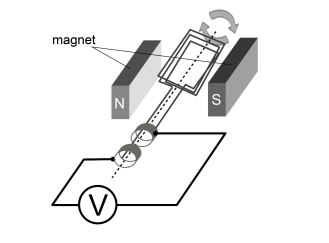# IMAT 2013 Q55 [AC Generators]

In an AC (alternating current) generator, a coil of wire rotates in a magnetic field.Which of the following would change the potential difference measured by the voltmeter in the system above?

1. Use more turns of wire in the coil
2. User thicker wire
3. Change the speed of rotation

A. 3 only
B. 1, 2 and 3
C. 1 and 2 only
D. 2 only
E. 1 and 3 only

Let us review each statement:

1. Using more turns of wire in the coil would increase the potential difference measured by the voltmeter in this system of an AC generator.

By having more and more loops of turns of the wire, the crests and troughs overlap the flux line at a faster rate or increasing the amount of times they cut through the magnetic field, and therefore the induced e.m.f will increase. This statement is therefore, correct.

1. Using a thicker wire in the coil would increase the potential difference measured by the voltmeter in this system of an AC generator.

The diameter or thickness of the wire could have an effect on it’s resistance on the current- but it has no relation to the induced potential difference in this system. Therefore, this answer is incorrect.

1. Changing the speed of rotation of the wire in the coil would increase the potential difference measured by the voltmeter in this system of an AC generator.

If the same coil of wire passed through the magnetic field, but it’s velocity or speed increases, the wires will cut the flux lines at a faster rate, therefore the induced e.m.f produced would also increase. Hence making this statement correct.

Our final answer will now be, considereing statements 1 and 3 are correct, E.

2 Likes

Doesn’t the increased thickness of wire increase resistance? If so, that would cause the voltage to drop, no?

Hi Karam,

So- no, in short. The thinner a wire is, the greater resistance it has; this is because a thinner wire has fewer electrons to carry an electric current. Using a thicker wire reduces resistance- it might be confusing but a good review of electricity and circuits will do you good.

Hope this helps.

Best,
Ahd

1 Like

Hi.
In the explanation above you said that thicker wires increase the potential difference by reducing the resistance.
But why does the answer say this view is wrong?
I don’t know which part is wrong.